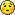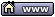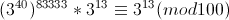flat assembler
Message board for the users of flat assembler.flat assembler > Heap > Calculating modular exponentiation manually
Author
 ThreadTmX

Joined: 02 Mar 2006
Posts: 820
Location: Jakarta, Indonesia
I once learnt this in the university, but already forgotten most of it. So I'd like to refresh my memory again.

Let's say you are asked to calculate (3 ^ 3333333) mod 100.
We can use this identity: ab mod N = (a mod N) (b mod N) mod N
Thus:
(3 ^ 3333333) mod 100
= (3 mod 100) (3^3333332 mod 100) mod 100
= 3 . (3^3333332 mod 100) mod 100
= 3 . 3 . 3 . 3 . 3 .... 3 mod 100

I'm stuck at that part.Are there any mathematical identities/formulas that can be used to simplify the calculation?03 Apr 2012, 06:42YONG

Joined: 16 Mar 2005
Posts: 8000
Location: 22° 15' N | 114° 10' E
(3 ^ 3333333) mod 100 = 2303 Apr 2012, 07:38YONG

Joined: 16 Mar 2005
Posts: 8000
Location: 22° 15' N | 114° 10' E
Note that 49^3 = 117649
So (49^3) mod 100 = 117649 mod 100 = 49
Hence (49^3n) mod 100 = (117649^n) mod 100 = (49^n) mod 100 ... (*)

(3 ^ 3333333) mod 100
= [3^(3333330+3)] mod 100
= [(3^3333330)(3^3)] mod 100
= {[(3^10)^333333]} mod 100
= [(59049^333333)(27)] mod 100
= [(49^333333)(27)] mod 100
= [(49^111111)(27)] mod 100
= ... | Repeatedly use (*) to reduce the number
= [(49)(27)] mod 100
= 1323 mod 100
= 2303 Apr 2012, 07:51TmX

Joined: 02 Mar 2006
Posts: 820
Location: Jakarta, Indonesia
(3 ^ 3333333) mod 100
= [3^(3333330+3)] mod 100
= [(3^3333330)(3^3)] mod 100
= {[(3^10)^333333]} mod 100
= [(59049^333333)(27)] mod 100

OK I understand this part. Now this is where the confusion begins:
= [(49^333333)(27)] mod 100

How do you get 49? 59049 mod 100?
So (a^b ) c mod N = (a mod N)^b c mod N?

And next:
= [(49^111111)(27)] mod 100
= ... | Repeatedly use (*) to reduce the number
= [(49)(27)] mod 100

How do you reduce [(49^111111)(27)] mod 100 into [(49)(27)] mod 100 ?
Are you implying that (a ^ b) c mod N = ac mod N?

Hmm.. this is quite hard to graspMaybe you have some readable articles on this topic?03 Apr 2012, 10:21MHajduk

Joined: 30 Mar 2006
Posts: 6027
Location: Poland
Alternative solution is presented on the picture attached below:03 Apr 2012, 11:48MHajduk

Joined: 30 Mar 2006
Posts: 6027
Location: Poland
In case you have any problems with displaying the aforementioned picture in your browser I can give you a link to the PDF file with this solution:

http://mikhajduk.houa.org/PDF/ModularExp.pdf

BTW, I spent some time writing this in LaTeX (for better readability) and I would like to know if TmX cares about it.03 Apr 2012, 18:36TmX

Joined: 02 Mar 2006
Posts: 820
Location: Jakarta, Indonesia
Hi MHajduk,

Thanks for providing another info. I've never heard of Euler's totient function before.
In general, I can understand your proof, except this part:How do you know both sides are congruent?BTW, I appreciate you wrote the proof in LaTeX, which is good for typesetting math formulasI wish this board could render LaTeX code properly.03 Apr 2012, 19:09MHajduk

Joined: 30 Mar 2006
Posts: 6027
Location: Poland
TmX wrote:
In general, I can understand your proof, except this part:How do you know both sides are congruent?You can multiply two congruences:

ifandthenSince we have proved that

(3^40)^83333 ≡ 1 (mod 100)

and we have a trivial congruence

3^13 ≡ 3^13 (mod 100)

so

(3^40)^83333 * 3^13 ≡ 3^13 (mod 100)

I hope this makes the whole calculation more understandable.03 Apr 2012, 19:19YONG

Joined: 16 Mar 2005
Posts: 8000
Location: 22° 15' N | 114° 10' E
TmX wrote:
...
Now this is where the confusion begins:
= [(49^333333)(27)] mod 100

How do you get 49? 59049 mod 100?
So (a^b ) c mod N = (a mod N)^b c mod N?

...

How do you reduce [(49^111111)(27)] mod 100 into [(49)(27)] mod 100 ?
Are you implying that (a ^ b) c mod N = ac mod N?
Note that 59049^333333 = (59049) (59049) ... (59049)
So, (59049^333333) mod 100
= [(59049 mod 100) (59049 mod 100) ... (59049 mod 100)] mod 100
= [(49) (49) ... (49)] mod 100
= (49^333333) mod 100

...
= [(59049^333333)(27)] mod 100
= {[(59049^333333) mod 100] [27 mod 100]} mod 100
= { {[(59049 mod 100)^333333] mod 100} (27) } mod 100
= {[(49^333333) mod 100] (27)} mod 100
= {[(49 mod 100)^333333] (27)} mod 100
= [(49^333333) (27)] mod 100
= [(49^111111) (27)] mod 100 | By (*)
= [(49^37037) (27)] mod 100 | By (*)
= [(49^37035) (49^2) (27)] mod 100
= [(49^12345) (49^2) (27)] mod 100 | By (*)
= [(49^4115) (49^2) (27)] mod 100 | By (*)
= [(49^4116) (49) (27)] mod 100
= [(49^1372) (49) (27)] mod 100 | By (*)
= [(49^1371) (49^2) (27)] mod 100
= [(49^457) (49^2) (27)] mod 100 | By (*)
= [(49^459) (27)] mod 100
= [(49^153) (27)] mod 100 | By (*)
= [(49^51) (27)] mod 100 | By (*)
= [(49^17) (27)] mod 100 | By (*)
= [(49^15) (49^2) (27)] mod 100
= [(49^5) (49^2) (27)] mod 100 | By (*)
= [(49^6) (49) (27)] mod 100
= [(49^2) (49) (27)] mod 100 | By (*)
= [(49^3) (27)] mod 100
= [(49) (27)] mod 100 | By (*)
= 1323 mod 100
= 2304 Apr 2012, 04:12TmX

Joined: 02 Mar 2006
Posts: 820
Location: Jakarta, Indonesia
@MHajduk
Ah, I forgot that part. Thanks for reminding me@YONG
Ah thank you. Actually this is the approach I am looking for. Sorry if I make myself unclear. MHajduk's approach, while shorter, is more 'advanced'. But anyway, both approaches are interesting.

Last edited by TmX on 04 Apr 2012, 20:30; edited 1 time in total04 Apr 2012, 16:06MHajduk

Joined: 30 Mar 2006
Posts: 6027
Location: Poland
TmX wrote:
Sorry if I make myself unclear. MHajduk's approach, while shorter, is more 'advanced'. But anyway, both approaches are interesting.
That's why we formulate theorems and use them. Well chosen theorem can radically shorten your calculations. Moreover, it ensures us that when some conditions are met, the aforementioned shortcut always leads to the proper results.04 Apr 2012, 18:09TmX

Joined: 02 Mar 2006
Posts: 820
Location: Jakarta, Indonesia
@MHajduk

I must be sleepy at that timeI think YONG's approach is good for visualizing the steps, while yours is for providing formal proof, without giving too much details.04 Apr 2012, 20:54Display posts from previous: All Posts1 Day7 Days2 Weeks1 Month3 Months6 Months1 Year Oldest FirstNewest FirstJump to: Select a forum Official----------------AssemblyPeripheria General----------------MainDOSWindowsLinuxUnixMenuetOS Specific----------------MacroinstructionsCompiler InternalsIDE DevelopmentOS ConstructionNon-x86 architecturesHigh Level LanguagesProgramming Language DesignProjects and IdeasExamples and Tutorials Other----------------FeedbackHeapTest Area

Forum Rules:
 You cannot post new topics in this forumYou cannot reply to topics in this forumYou cannot edit your posts in this forumYou cannot delete your posts in this forumYou cannot vote in polls in this forumYou can attach files in this forumYou can download files in this forum